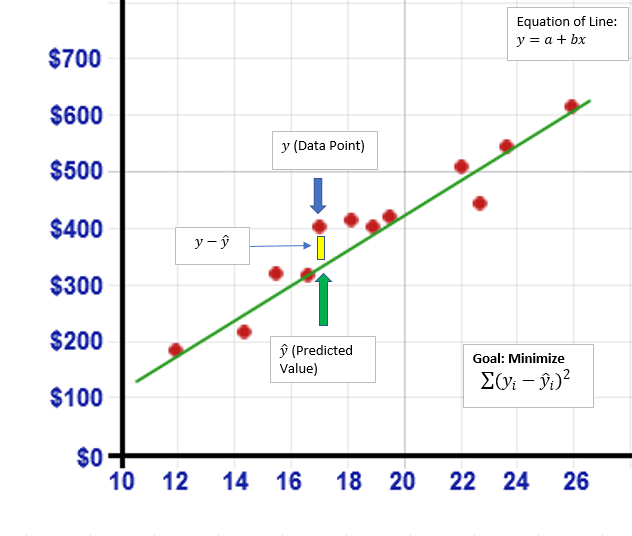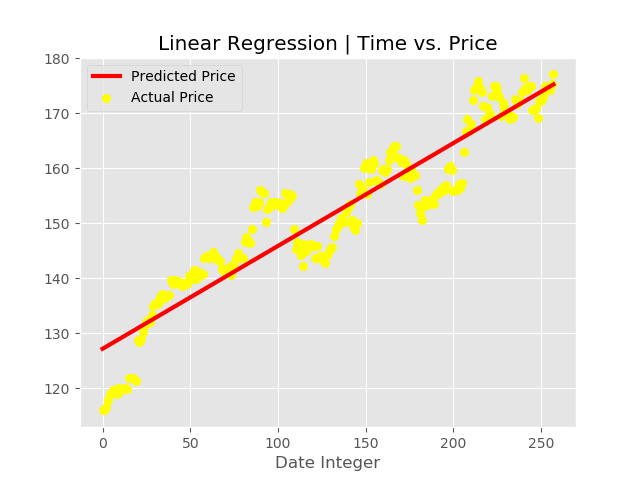# python用线性回归预测股票价格的实现代码

更新时间：2019年09月04日 14:48:38   作者：qq_19600291我要评论

y = a + bx

• Y =预测值或因变量
• b =线的斜率
• x =系数或自变量
• a = y截距```from matplotlib import style

from sklearn.linear_model import LinearRegression

from sklearn.model_selection import train_test_split

import quandl

import datetime

style.use('ggplot')

#Dates

start_date = datetime.date(2017,1,3)

t_date=start_date, end_date=end_date, collapse="daily")

df = df.reset_index()

prices = np.reshape(prices, (len(prices), 1))```

```', linewidth=3, label = 'Predicted Price') #plotting the line made by linear regression

plt.title('Linear Regression | Time vs. Price')

plt.legend()

predicted_price =regressor.predict(date)``````et

xtrain, x , ytrain)

#Train

plt.title('Linear Regression | Time vs. Price')

#Test Set Graph

plt.scatter(xtest, ytest, color='yellow', label= 'Actual Price') #plotting the initial datapoints

plt.plot(xtest, regressor.predict(xtest), color='blue', linewidth=3, label = 'Predicted Price') #plotting

plt.show()```